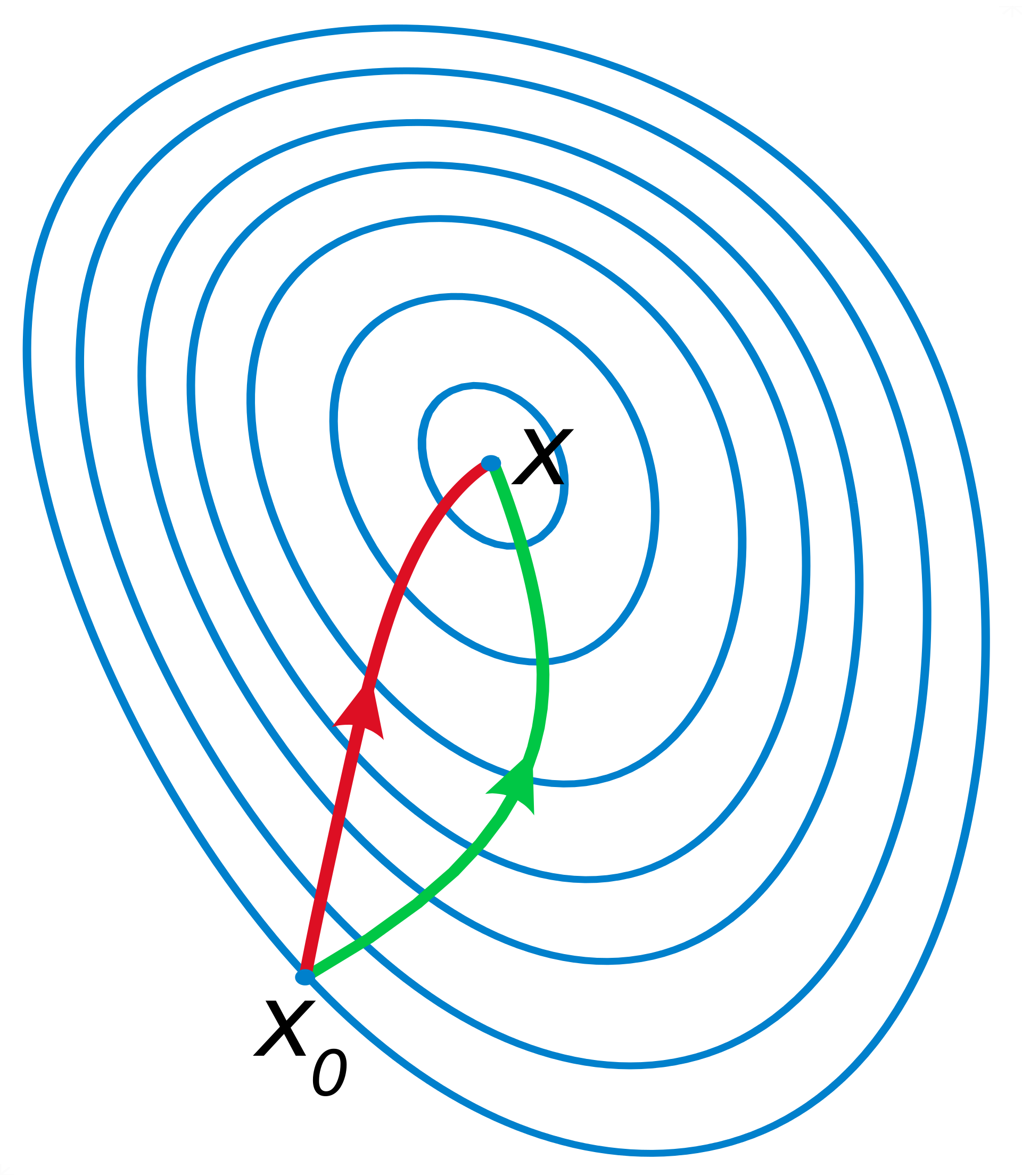# 机器学习笔记-梯度下降法和牛顿法

## 梯度下降法

$f(x^{k+1})$$x^{k}$ 处一阶泰勒展开得： $f(x^{k+1}) = f(x^{k} + \Delta t) \approx f(x^{k}) + f'(x^{k}) \Delta t$ ，按梯度方向不断迭代逼近可取 $\Delta t = - \lambda \cdot f'(x^k)$ ，其中 $\lambda$ 为步长；

## 牛顿法

$f(x^{k+1})$$x^k$ 处二阶泰勒展开得： $f(x^{k+1}) = f(x^k + \Delta t) \approx f(x^k) + f'(x^k) \cdot \Delta t + \frac{1}{2} \cdot f''(x^k) \cdot (\Delta t)^2$ ，通常将一阶导和二阶导分别记为 $g$$h$$f(x^{k+1}) \approx f(x^k) + g \cdot \Delta t + \frac{1}{2} \cdot h \cdot (\Delta t)^2$ ，因为要使 $f(x^{k+1})$ 取得极小值，则令 $\frac{ \partial ( g \Delta t + \frac{1}{2} h \Delta t^2 ) } {\Delta t} = 0$ ，得到 $\Delta t = - \frac{g}{h}$ ，则 $x^{k+1} = x^{k} - \frac{g}{h}$ ，按二阶梯度方向不断迭代逼近；

## 区别## 参考文献

1. 梯度下降法与牛顿法比较

2. 《统计学习方法》附录A 与 附录B